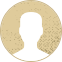Top Special Offer! Check discount

Get 13% off your first order - useTopStart13discount code now!

# Intertemporal choice model

67 views 3 pages ~ 677 words
Get a Custom Essay Writer Just For You!

Experts in this subject field are ready to write an original essay following your instructions to the dot!

A method used in advanced macroeconomics called the intertemporal choice model is used to describe the choice a consumer makes between consumption in the present and consumption in the future. According to the Absolute Income Hypothesis, a person's consumption for the current year is only dependent on their present income. As long as individuals are making decisions, they will always take the present and the future into account. Therefore, the consumer will always have to decide between current consumption and consumption in the future.

Keynes examined a rational consumer who lived in two periods to determine the trade-off between consumption in the two. Consumption in first phase is denoted by CI while that in the second phase is denoted by C2.

Y1 = 20,000

Y2 = 25,000

r = 5%

In period one, the consumption is constrained by:

C1+ S1 = Y1

The household saves in the first period to consume in the second period. The consumption constraint in the second period is

C2 = Y2 + (1+r) S1

The consumer will consume everything in the second period and therefore, do not save.

Therefore, the intertemporal budget constraint which the consumer faces is as shown below,

(1)

(2)

Thus, substituting equation (1) into equation (2) we obtain,

(3)

Rearranging equation (3) above we get,

(4)

Dividing equation (4) by we have,

(5)

Replacing the value of in equation (5) we get,

(6)

Equation (6) represents the budget constraint of the consumer whose slope or gradient gives the marginal rate of substitution of.

Saving

Borrowing

0

In the graph below, the optimal consumption point is at point O. Since the household is rational, the optimal consumption point will be at point O.

O

0

If at the current rate of 5% it is optimal for the households to borrow, then it implies that C1 is greater than 20,000. The household will then borrow the maximum amount possible in order to meet their demand.

G

Borrowing

0

F

G

0

At the point G, the marginal rate of substitution is low since C1 is high while C2 is low. This means that, the households will require fewer amounts of C2 to sacrifice an extra amount of C1 to remain at the same utility level as shown by the time indifference curve. This curve shows the various combinations of C1 and C2 that gives the same satisfaction.

Part (b)

Increasing the market interest from 5% to 15% will lead to C1 being greater than Y1. Therefore, since the consumers are rational and assuming there is no borrowing constraint, the households will decide to borrow so as to consume in C1. This situation causes the budget constraint to tilt to the left as shown in the graph below.

F

G

0

The consumption in period two in the above graph will reduce to increase the consumption in period one. The optimal consumption point in both periods will be on a lower indifference curve giving less satisfaction as compared to the optimal point when the market interest rate is at 5%.

Part (c)

Supposing that the government imposes a subsidy on the interest rate to encourage household to save, the consumer will save a maximum of C1 in the first period in order to benefit from the increased interest rates on savings. In this case, the borrowing constraint is non-binding since the user is a saver and does not borrow. In the graph below, the household would wish to consume at point D because they are rational; but since borrowing constrains them, they will be forced to consume at point E.

Saving

E

Borrowing

D

0

The increase in interest rates on savings will decrease the consumption rate in the first period of the households thus bringing the issue of substitution and income effect. Increase in interest rates will tend to tilt the budget constraint to the right hence making it to be more steeper as shown below.

B

A

0

The household will substitute consumption in the current period for the future consumption in period two. The consumer is better off at point B than at A since he or she is on a higher indifference curve indicating greater satisfaction. Therefore, the policy of subsidizing interest rates is effective in encouraging households to save.

April 13, 2023
Category:
Subject area:
Number of pages

3

Number of words

677

48Rate:

4.6

Expertise Decision
Verified writer

JakeS has helped me with my economics assignment. I needed an urgent paper dealing with Brexit. JakeS has been awesome by offering an outline with ten sources that have been used. It helped me to avoid plagiarism and learn more about the subject.

Hire Writer

This sample could have been used by your fellow student... Get your own unique essay on any topic and submit it by the deadline.

Eliminate the stress of Research and Writing!

Hire one of our experts to create a completely original paper even in 3 hours!